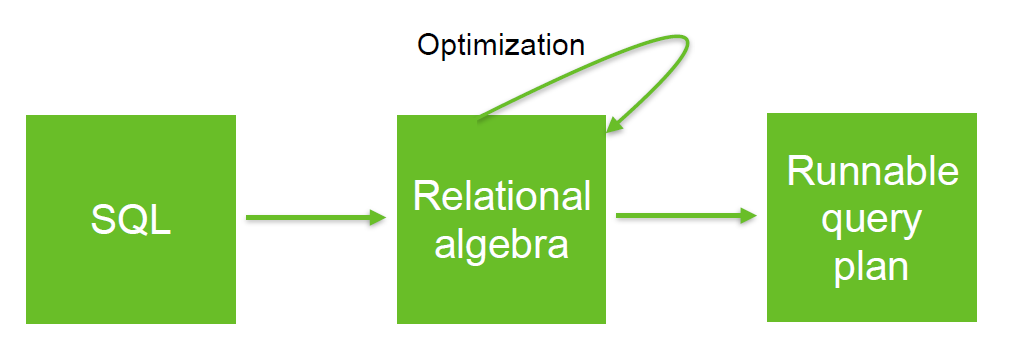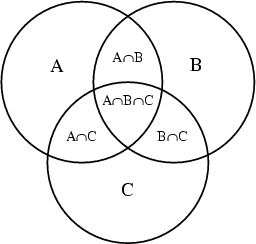# Relational Algebra - Intersect operator (intersection)

intersect (or intersection) is a relational operator that returns the intersection of two relation A and B, denoted by A ∩ B.

An intersection is defined as all tuples (rows) that are present in both of two union-compatible (same columns and same type) relation A and B.

The following definition are also equivalent.

• all elements of A that also belong to B
• all elements of B that also belong to A

## Syntax

### Minus

A derived operator using minus (-) where the minus expression $( R1 - R2 )$ returns anything that is only in R1

$$\Large R1 \cap R2 = R1 - ( R1 - R2 )$$

### Join

• Derived using join

$$\Large R1 \cap R2 = R1 \times R2$$

## Documentation / Reference

Discover MoreRelational Algebra - Expression and Operators

Relational algebra is based upon the fact that you can pass tabular data through a set of data operators (select, filter, join, sort, union, etc.) in a algebraic structure. It means that: the output...Relational Operator - Equi-joins

An Equi-join is a join where the condition (predicate) is an equality. theta join An equi-join links two relations (tables, ...) on common values in a column in relation 1 with a column in relation...SQL - Joins (Home)

in SQL. A join is a SQL clause statement which define the set operation such as: intersection, union, that the database must perform between two or more relation (table, view, query, ...). The...Sql - Intersect

in SQL